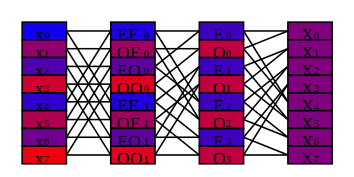Top: FFT on an FPGA

Previous: Introduction

# Derivation of Decimation-in-Time Fast Fourier Transform

I chose to implement the decimation-in-time (DIT) form of a FFT not because I thought it would result in an efficient implementation but rather because the algorithm seemed conceptually simpler. I have favored simplicity over efficiency since this is my first verilog design.

The definition of a discrete fourier transform (DFT) is

\begin{equation*} X_k = \sum_{n=0}^{N-1}{x_n e^{-2 \pi i k n/N}} \end{equation*}

where $$x_n$$ is a sequence of length $$N$$ and $$X_k$$ is the fourier transform of this sequence.

The most common FFT algorithm is the Cooley-Tukey FFT algorithm which takes advantage of the fact that a discrete fourier transform (DFT) can be expressed in terms of a combination of two smaller DFTs.

Let $$e_n$$ be the even components of $$x_n$$ ($$e_n = x_{2n}$$).

Let $$o_n$$ be the odd components of $$x_n$$ ($$o_n = x_{2n+1}$$).

Let $$E_k$$ and $$O_k$$ be their DFTs.

\begin{equation*} \begin{aligned} X_k & = \sum_{n=0}^{N-1}{x_n e^{-2 \pi i k n/N}} \\ & = \sum_{n=0}^{N/2-1}{x_{2n} e^{-4 \pi i k n/N}} + \sum_{n=0}^{N/2-1}{x_{2n+1} e^{-2 \pi i k (2n+1)/N}} \\ & = \sum_{n=0}^{N/2-1}{e_n e^{-2 \pi i k n/(N/2)}} + e^{-2 \pi i k/N}\sum_{n=0}^{N/2-1}{o_n e^{-2 \pi i k /(N/2)}} \\ & = E_k + e^{-2 \pi i k/N} O_k \end{aligned} \end{equation*}

Since DFTs wrap around, $$E_k = E_{k-N/2}$$, and $$e^{ix} = -e^{i(x+\pi)}$$, we can write $$X_k$$ as:

\begin{equation*} \begin{aligned} X_k & = E_k + e^{-2 \pi i k/N} O_k \\ X_{k+N/2} & = E_k - e^{-2 \pi i k/N} O_k \end{aligned} \end{equation*}

Equations 1 and 2

so that we only need to consider $$0 < k \le N/2$$ to generate all of $$X_k$$.

Using these equations the DFT is calculated in a series of stages where in each stage we calculate a new set of N complex numbers from the N complex number of the previous stage.Figure 1: Schematic of DFT of size 8.

Figure 1 shows a schematic of the calculation of a DFT of size 8. The column of rectanges on the left represent $$x_n$$, the input data to the DFT (the zeroth stage). The column of rectangles on the right represent $$X_k$$, the output of the DFT (the third stage). In between are two intermediate stages. The data in the second stage is labelled with $$E_k$$ and $$O_k$$ so that we can see how to apply equations 1 and 2 to calculate the third stage from the second. The data in the first stage is labelled with $${EE}_k$$, $${OE}_k$$, $${EO}_k$$, and $${OO}_k$$, where $${OE}_k$$ is the fourier transform of $${oe}_n$$ which is the even components of $$o_n$$, the odd components of $$x_n$$. We can calculate $$E_k$$, using equations 1 and 2, from $${EE}_k$$ and $${EO}_k$$, and similarly we calculate $$O_k$$ from $${OE}_k$$ and $${OO}_k$$. The lines in Figure 1 show the dataflow from one stage to the next.

In general, stage $$s$$ is an interleaving of $$S=N/2^s$$ transforms of length $$M=N/S$$. Let $$P_{s,i}$$ be component $$i$$ of stage $$s$$. Let $$X_{s,j,k}$$ be component $$k$$ of transform $$j$$ in stage $$s$$. So in Figure 1 $$E_3$$ is component 6 (we index from 0) of stage 2, $$P_{2, 6}$$, and is also component 3 of transform 0 of stage 2, $$X_{2, 0, 3}$$.

\begin{equation*} X_{s,j,k} = P_{s,kS+j} \end{equation*}

The DFT $$X_{s,j,k}$$ ($$k$$ is position in the DFT) was generated by combining two DFTs from the previous stage, $$X_{s-1,j,k}$$ and $$X_{s-1,j+S,k}$$. We then have,

\begin{equation*} \begin{aligned} X_{s,j,k} & = X_{s-1,j,k} + e^{-2 \pi i kS/N} X_{s-1,j+S,k} \\ P_{s,kS+j} & = P_{s-1,2kS+j} + e^{-2 \pi i kS/N} P_{s-1,2kS+S+j} \\ P_{s,kS+j} & = P_{s-1,2kS+j} + T_{kS} P_{s-1,2kS+S+j} \end{aligned} \end{equation*}

Equation 3

where $$T_{n} = e^{-2 \pi i n/N}$$.

And similarly for the second half of P we have,

\begin{equation*} P_{s,kS+j+N/2} = P_{s-1,2kS+j} - T_{kS} P_{s-1,2kS+S+j} \end{equation*}

Equation 4

This completes the derivation of the algorithm and in the next section we'll describe the verilog implementation.